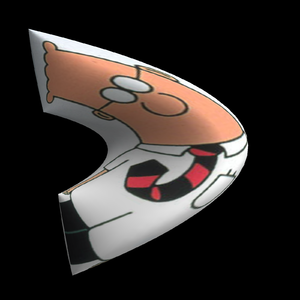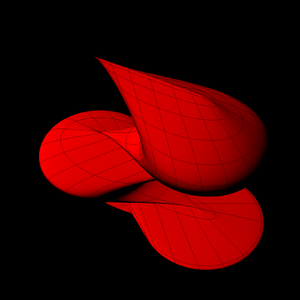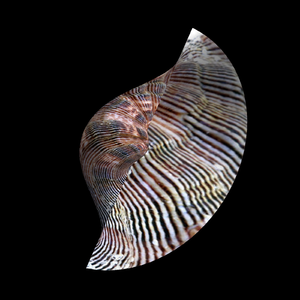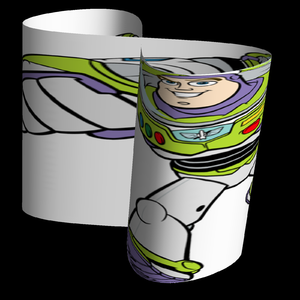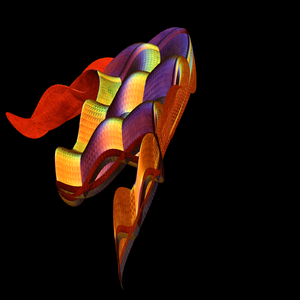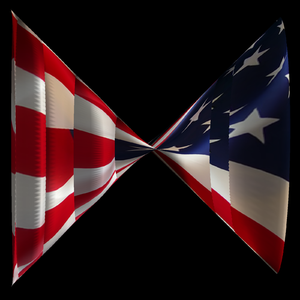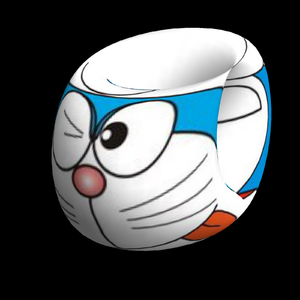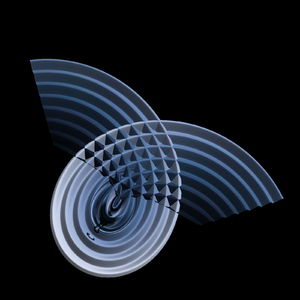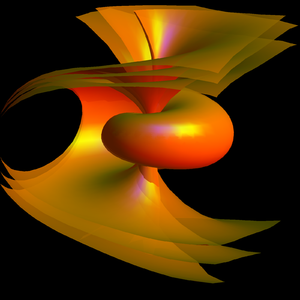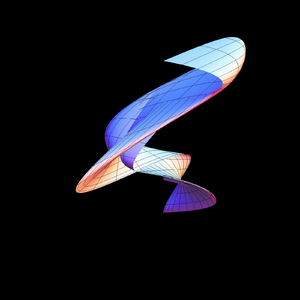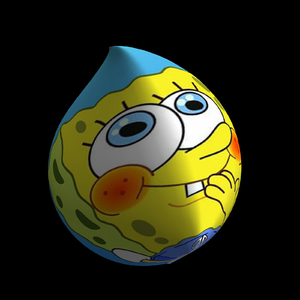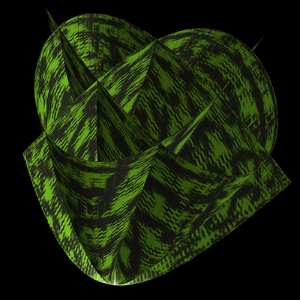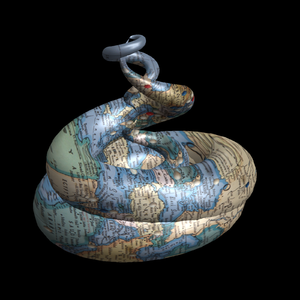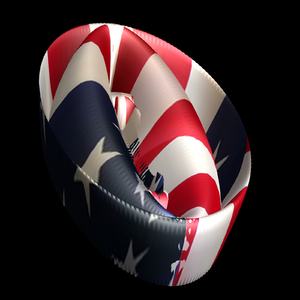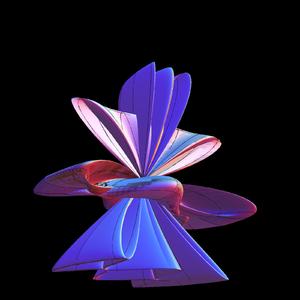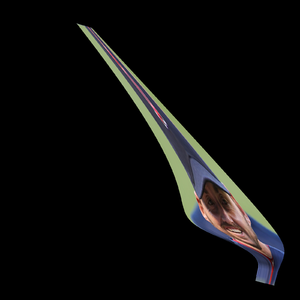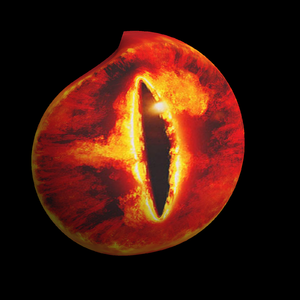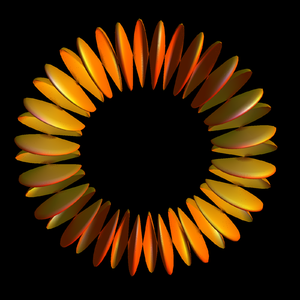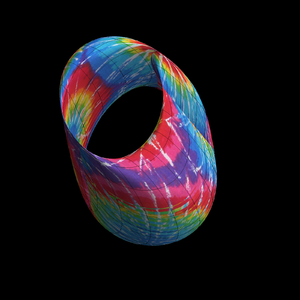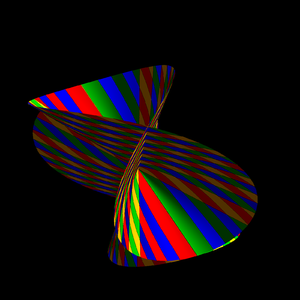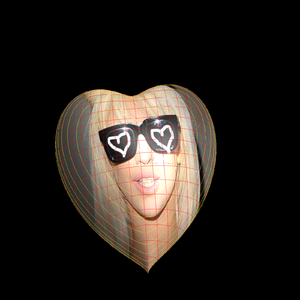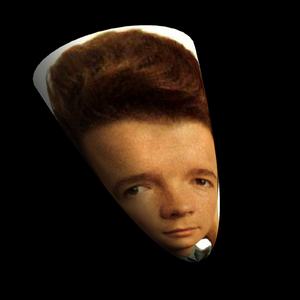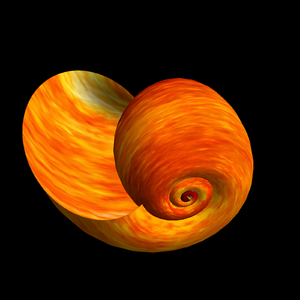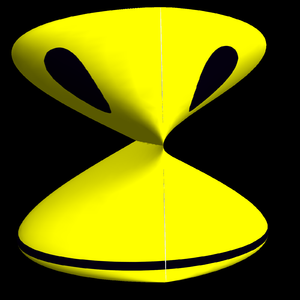Problem A: Produce your own parametrized surface. You can use any tool. Here are some ideas in a Mathematica notebook (right click here) save it and open it in Mathematica.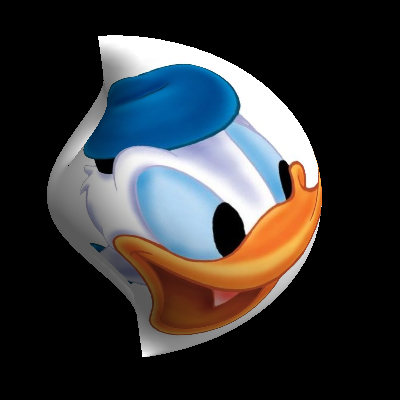Donald's picture in the parameter plane is mapped onto the surface. You can play with parameters like "Mesh->True" (place the mouse over Donald to see the grid curves). Here is the Mathematica code for the picture to the left:
```picture = Import["http://www.courses.fas.harvard.edu/~math21a/donald.jpg"];
ParametricPlot3D[(2+Sin[t]) {Cos[t] Sin[s], Sin[t] Sin[s],Cos[s]},
{s,0,Pi},{t,0,2Pi}, Lighting -> "Neutral", Mesh -> False, PlotPoints->40,
TextureCoordinateFunction -> ({#5, #4} &), PlotStyle -> {Texture[picture]},
ViewPoint -> {-3.25,-0.9,-0.2},ViewVertical->{-0.64,-0.05,-0.9},
Axes -> False, Boxed -> False, PlotRange->All]
```
You see that parametrizations are very useful in graphics. It is used in computer games, to make surfaces look realistic, in google earth to texture the buildings and ground.

Here is an example, where the texture is one of the built in Metal textures of Mathematica. Here is the code:
```f[t_] := {Sin[t], Sin[3 t], Sin[2 t]};
ParametricPlot3D[f[t]+f[s], {t,0,2Pi}, {s,0,2Pi},
TextureCoordinateFunction -> ({#4,#5} &), AspectRatio -> 1,
PlotStyle -> Directive[Texture[ExampleData[{"ColorTexture", "Metal1"}]]],
ViewPoint->{-1.8,-2.6,-1.17},ViewVertical->{-1,0,-0.1},PlotPoints->100,
AspectRatio->1,Boxed->False,Mesh-> False, Axes -> False];
```
The animation to the left plays with the parametrization and takes r(t,s) = c f[t] + (2-c) f(s) for various c. The example code above has c=1, where r(t,s) = f[t] + f[s]
If you should not have Mathematica ready, don't sweat it. Here are some online tools, with which you can solve Problem A too: these tools plot spherical surfaces rho=f(theta,phi) which are parametrized as follows
```r(u,v) = f(u,v) < cos(u) sin(v), sin(u) sin(v), cos(v) >
```
The function f(u,v) gives the distance from the origin as a function of the angles u=theta, v=phi. The task of problem A is to render a surface of your choice and print it out. If you use Mathematica, you can of course modify some of the templates above. You can mail the notebook or modified code to knill@math.harvard.edu. We might add some your pictures here.